output.to from Sideway
Draft for Information Only

# Content

```Equivalent Couples  Non-Parallel Coplanar Couples Transformation ```

# Equivalent Couples

Equivalent couples can also be formed by the combination of simple transformations.

## Non-Parallel Coplanar Couples Transformation

Two coplanar couples are equal when the force couples can be transfromed by making use of principle of transmissibility, parallelogram law of force decomposition and simple couple translation and couple rotation in space.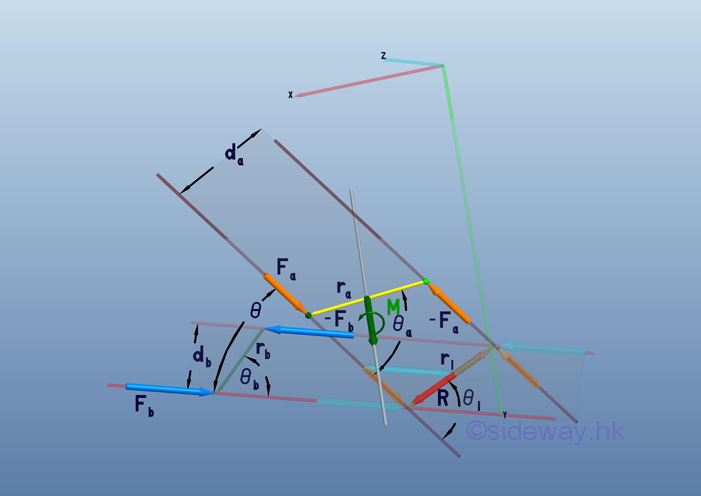By the principle of transmissibility, both force vectors,  Fa and  Fb  can be translated along its line of action. For non parallel coplanar force couples, force Fa can be resolved into force Fb and force along moment arm r by force decomposition.

The effective moment of both force couples can be expressed as: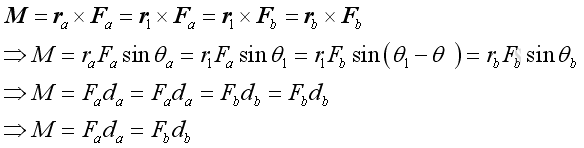By principle of transmissibility, imply moment arms ra and rb can be expressed in terms of r1: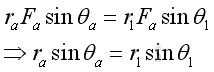and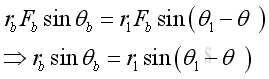Geometrically, prependicular distances da and db can be expressed as: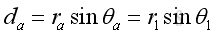and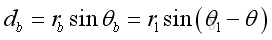Imply the prependicular distance db can be expressed in terms of da as: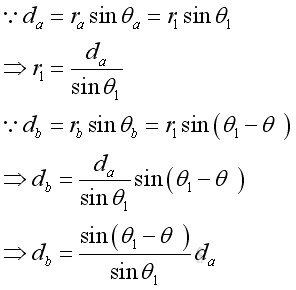By the sine rule, imply force Fb can be expressed in terms of Fa: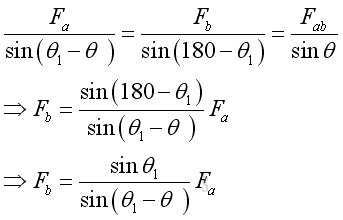Therefore the moment M is: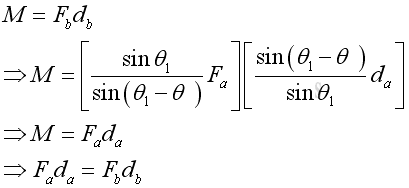By the sine rule and the cosine rule, the angle θ1 between force Fa and the moment arm r can be expressed in terms of magnitude of forces Fa and Fb , and the angle between them: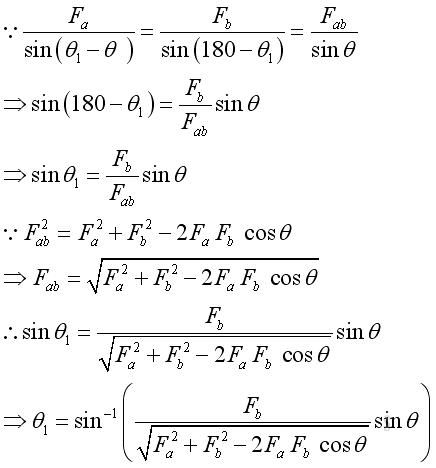The moment vector M can be expressed as: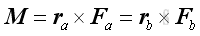For non parallel coplanar force couples, force Fa can be resolved into force Fb and a vector difference force R by force decomposition. Imply: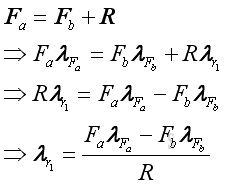The magnitude of the vector difference force R can be expressed as: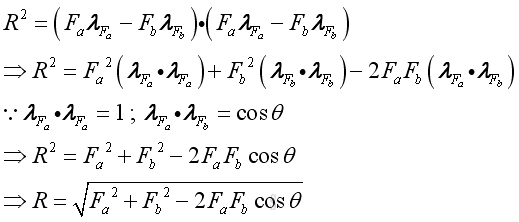Therefore the direction vector of the vector difference force R is: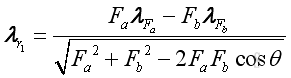Since two force couples are equivalent and can have the same moment arm vector r1 along  the direction vector of the vector difference force R after transformation, the cross product of moment arm vector r1 and forces Fa and Fb is the same. Proof: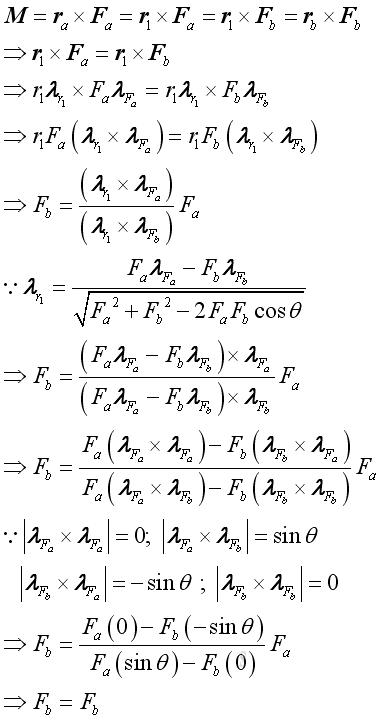Therefore the forces of couples can be expressed as :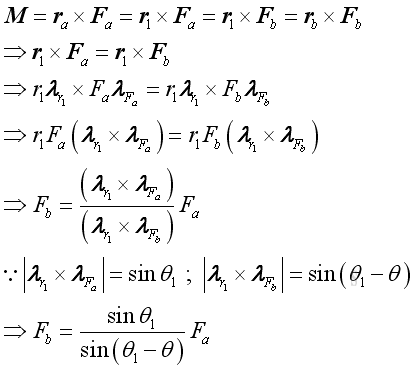To simplify the vector analysis, the non parallel coplanar force couples transformation can be reduced to 2D rotation by transformation through aligning the transformation axis normal to plane of force couple at the intersection of forces Fa and Fb to the z axis of the rectangular coordinate.  imply: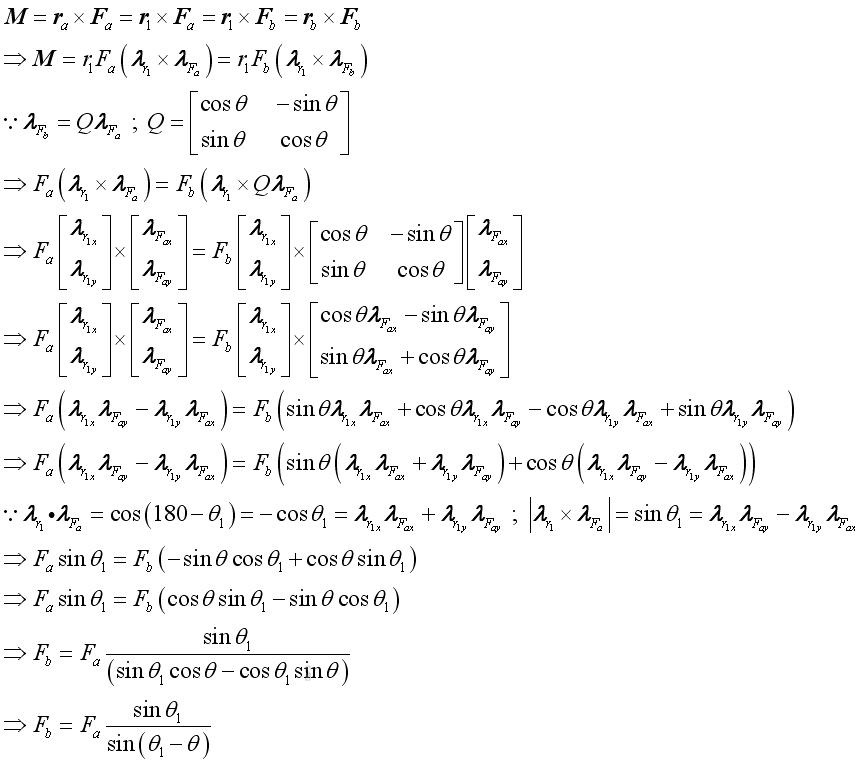The prependicular distance of the moment of a force couple is equal to the magnitude of the cross product of the moment arm vector of the force and the direction vector of the force. When two non parallel coplanar force couples are equivalent, the moment arms of the two force couples can be transformed to the same moment arm by the principle of transmissibility. Imply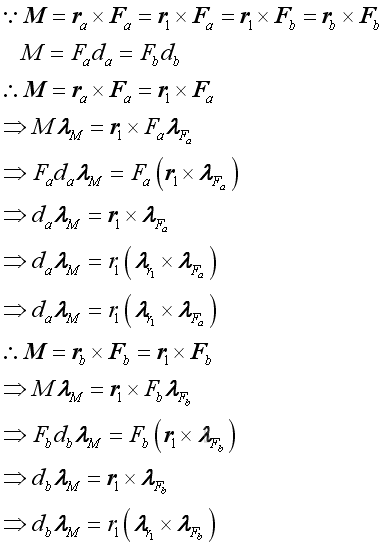Therefore the two prependicular distances of the two force couples can be related by the magnitude of moment arm.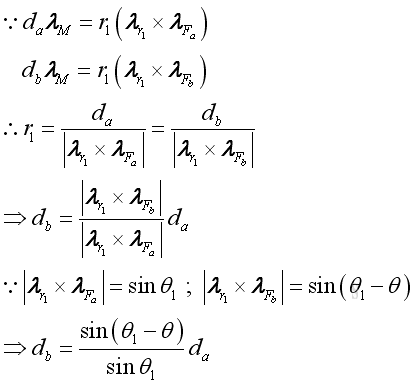Therefore the moment M is:ID: 110600008 Last Updated: 6/30/2011 Revision: 0 Ref:References

1. I.C. Jong; B.G. rogers, 1991, Engineering Mechanics: Statics and Dynamics
2. F.P. Beer; E.R. Johnston,Jr.; E.R. Eisenberg, 2004, Vector Mechanics for Engineers: StaticsHome 5

Management

HBR 3

Information

Recreation

Culture

Chinese 1097

English 339

Computer

Hardware 249

Software

Application 213

Latex 52

Manim 205

KB 1

Numeric 19

Programming

Web 289

Unicode 504

HTML 66

CSS 65

SVG 46

ASP.NET 270

OS 429

Python 72

Knowledge

Mathematics

Algebra 84

Geometry 34

Calculus 67

Engineering

Mechanical

Rigid Bodies

Statics 92

Dynamics 37

Control

Natural Sciences

Electric 27